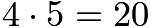# May 2017 Brain Puzzler Solution

Q: How many three-digit numbers satisfy the property that the middle digit is the average of the first and the last digits?

A: 45

With 1 as a middle digit, there are two three digit numbers whose middle digit is the average of the first and last digits:  111 and 210

There are 4 numbers with 2 as a middle digit: 123, 220, 321, 420
There are 6 numbers with 3 as a middle digit: 135, 234, 333, 432, 531, 630
There are 8 numbers with 4 as a middle digit: 147, 246, 345, 444, 543, 642 741, 840
There are 9 numbers with 5 as a middle digit: 159, 258, 357, 456, 555, 654, 753 852, 951
There are 7 numbers with 6 as a middle digit: 369, 468, 567, 666, 765, 864, 963
There are 5 numbers with 7 as a middle digit: 579, 678, 777, 876, 975
There are 3 numbers with 8 as a middle digit: 789 888, 987
There is 1 number with 9 as a middle digit: 999

Total: 2 + 4 + 6 + 8 + 9 + 7 + 5 + 3 + 1 = 45

Another way to solve this problem is to note that the middle digit is half the sum of the first and third digit so the sum must be even to result in a whole number when divided by 2.  To get two numbers to add to an even number they must be both odd or both even. Notice this in the list of 3 digit numbers above!

If both the first digit and the last digit are odd, then 1, 3, 5, 7, or 9 are choices for each of these digits, and there are$5\cdot5=25$ numbers in this case.
If both the first and last digits are even, then 2, 4, 6, 8 are choices for the first digit and 0, 2, 4, 6, 8 for the third digit. There are$4\cdot5=20$ numbers here.  25 + 20 = 45